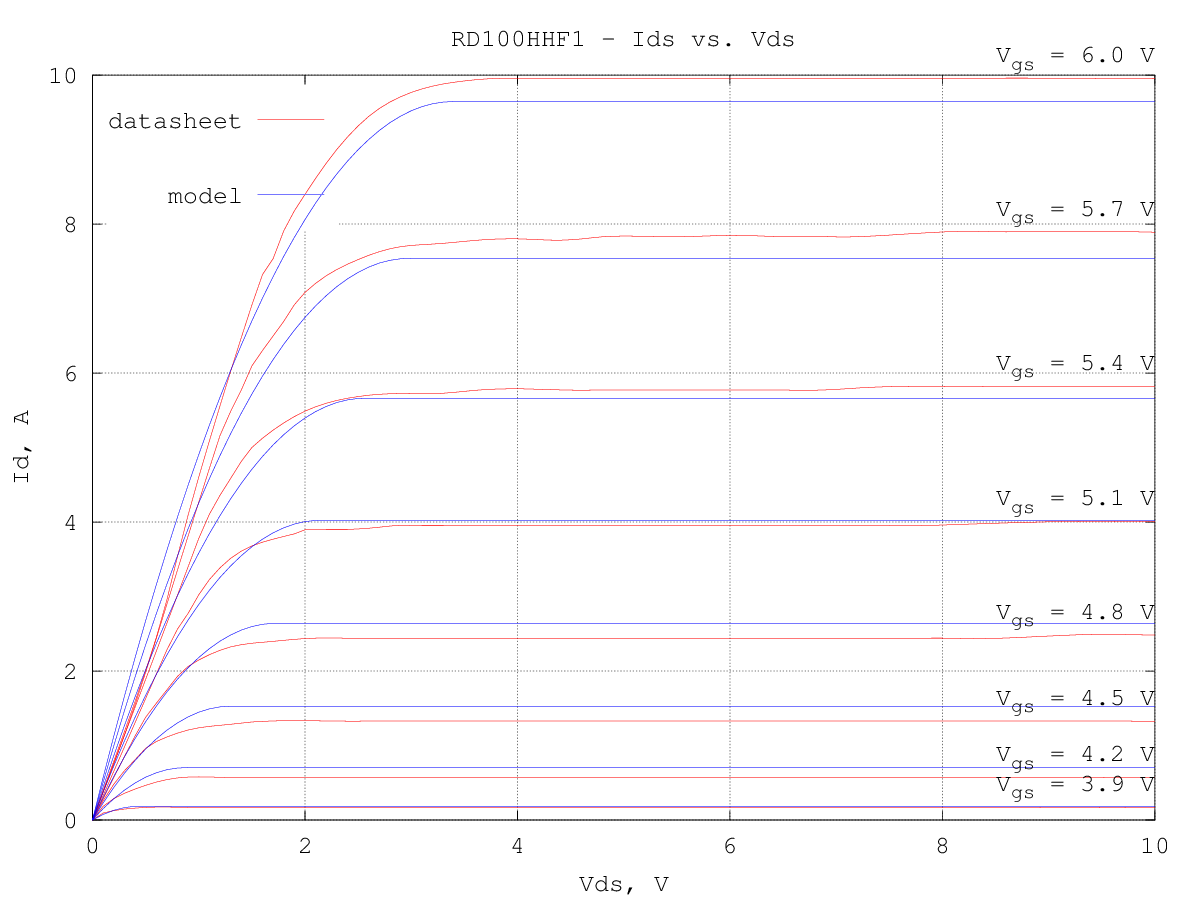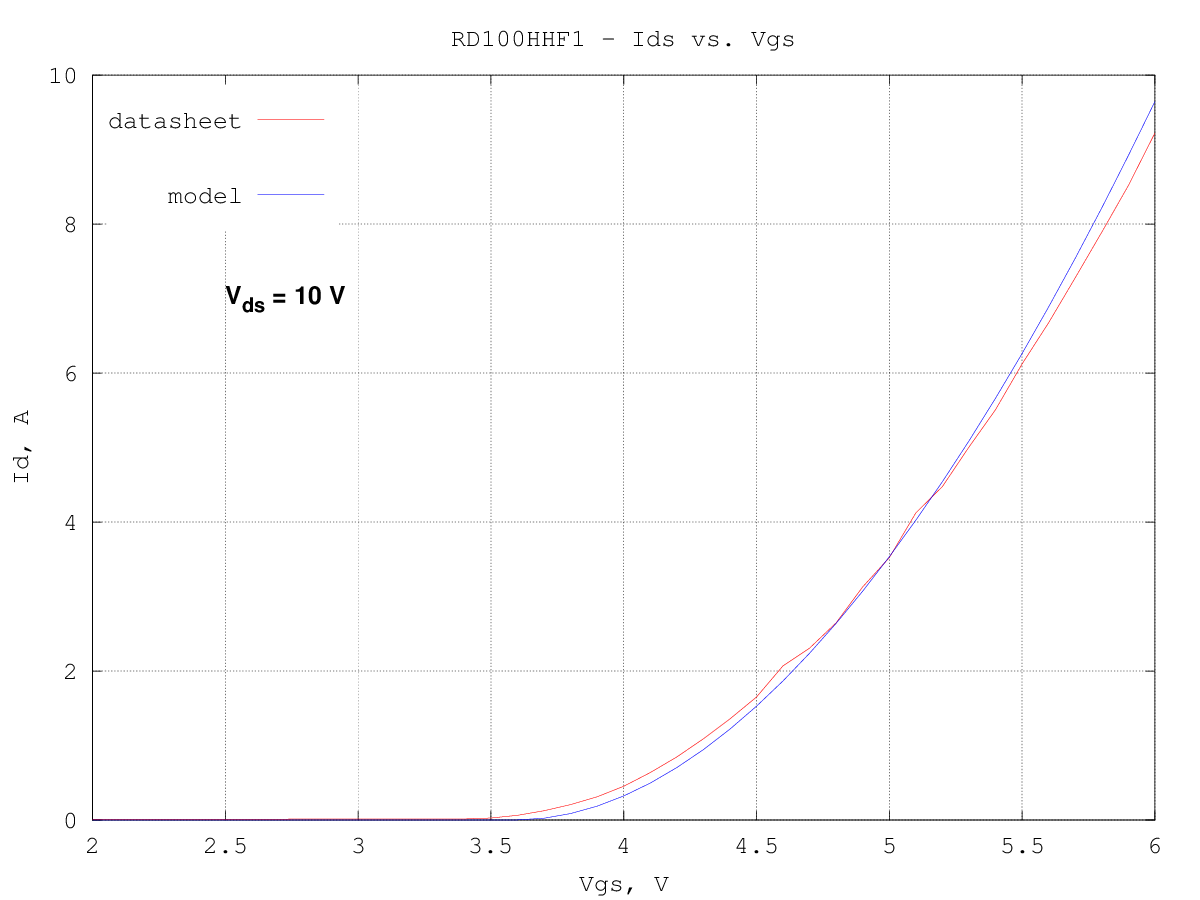# Mitsubishi RD100HHF1 LDMOS LTspice model

The device has been modeled using the LTspice VDMOS model, since it is well suited also for LDMOS devices and contains only few of parameters which can be guessed from the scarce data available from the datasheet.
The static (drain current) and dynamic (capacitances) characteristics were separately optimized, starting from a first guess extracted from the curves in the datasheet. The optimized model description is:

.model RD100HHF1 VDMOS(Rg=0.576 Rd=0 Rs=21m Vto=3.59 Kp=3.96 Lambda=0 mtriode=0.692 subthres=1.07m Cgdmax=58.5p Cgdmin=6.34p Cgs=250.5p Cjo=344.7p M=0.3333 Vj=1.673)

the quality of the fit is relatively good even if the VDMOS model, being targeted mainly at switching circuits applications, does not have many parameters to optimize. The gate-drain capacitance model fit is the less accurate.
Another problem found during the VDMOS model extraction is that even the datasheet graphs are not entirely consistent, as the Ids vs. Vds curves do not exactly agree with the Ids vs. Vgs curves, so the model extracted tries to be a best compromise between the two curves...

Here below is a graph of the drain current vs. the drain voltages for several values of the gate voltage; the fit is relatively good in the saturation region but only fair in the linear region:The Ids vs. Vgs curve fit, for a fixed drain voltage of 10 V, fits quite well the entire range of the datasheet curve:Input (Ciss) and output (Coss) capacitances are well modeled, while the reverse transfer capacitance (Crss) is quite off, as the voltage dependency is not matching with the one implemented in the LTspice model: